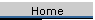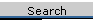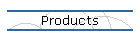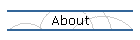Apr/May/June 2002

We're back for the 8th edition of our newsletter!  We hope everyone had a wonderful spring (or I guess Fall in the Southern hemisphere).

It has been a long journey for us, but we see the end of the tunnel for the Inference Engine Component Suite v2.0.  There is more information on the release later in the edition.  In addition, our main topic this time is the powerful fuzzy expressions you can create in IECS v2.0.  Also, we give a couple of great tips.  One to use a little known feature of the IECS v2.0EEP.  The other is a continuation of a tip from last time, instead of writing rules to create aggregate facts, this time we will be writing a function to do it for us.

from the web site at
http://www.riversoftavg.com/articles_&_tips.htm

Contents

Article: About Fuzzy Expressions in IECS v2.0
News: Version 2 Coming in July
News: RiverSoftAVG is a Borland Technology Partner
Tip: Using Current Rulename variable for debugging
Tip: How to write a function to collect information about some facts

Note: You received this newsletter because you are an owner of a RiverSoftAVG product.  If you received this newsletter by mistake or if for any reason you wish to not receive any future mailings from RiverSoftAVG, just reply to this message informing us so.  We apologize for any intrusion.

Article: About Fuzzy Expressions in Inference Engine Component Suite Version
2.0 EEP

One of the major features of the IECS v2.0 is the fuzzy logic.  We have discussed before, in general, the fuzzy logic support in v2.0.  In this
article, we are going to talk in more detail about what fuzzy expressions you can make, how you can combine, and how you can modify fuzzy sets.  The Fuzzy Logic in
the IECS has a full-featured syntax for creating fuzzy expressions or calculations.  Fuzzy expressions create fuzzy sets, which are created by
defining the XY value singletons that specify the shape of the fuzzy set, by functions that return a fuzzy singletons shape, or by modifying other fuzzy
sets through operations and hedges.  Note that fuzzy sets are also called fuzzy terms or fuzzy values in the IECS.

The IECS allows you to define or modify fuzzy sets or values in the following ways:Define Fuzzy Sets using SingletonsDefine Fuzzy Sets using Scalars or FunctionsModify Fuzzy Sets using HedgesDefine Fuzzy Sets using other Fuzzy Sets and Operations
For our discussion, we are going to assume that a fuzzy variable, temperature, has been defined like so:

> (ppdeftype temperature)

(deftype temperature extends (type FUZZY)
(range 0.0000 100.0000)
(values
(term low ((0.0000 1.0000) (100.0000 0.0000) ))
(term medium (tri 0.0000 50.0000 100.0000))
(term high ((0.0000 0.0000) (100.0000 1.0000) ))
))

which, when graphed, looks like so:

> (plot-fuzzy-value t *+- n n temperature)

Fuzzy Variable: temperature

Fuzzy Values: medium(*) high(+) low(-)

1.0000--                       *                       +

0.9500  --                    * *                    ++

0.9000    ---               **   **               +++

0.8500       --            *       *            ++

0.8000         ---        *         *        +++

0.7500            --     *           *     ++

0.7000              ---**             **+++

0.6500                *--             ++*

0.6000               *   ---       +++   *

0.5500              *       --   ++       *

0.5000            **          ---          **

0.4500           *          ++   --          *

0.4000          *        +++       ---        *

0.3500         *       ++             --       *

0.3000       **     +++                 ---     **

0.2500      *     ++                       --     *

0.2000     *   +++                           ---   *

0.1500    *  ++                                 --  *

0.1000  **+++                                     ---**

0.0500 *++                                           --*

0.0000++                                               -

|----|----|----|----|----|----|----|----|----|----|

0.00     20.00     40.00     60.00     80.00    100.00

Universe of Discourse: From 0.00 to 100.00

Define Fuzzy Sets using Singletons

The most simplistic way to define fuzzy sets is to define a singletons vector of XY value pairs.  The singletons vector defines a vector of XY value pairs that control the shape of the fuzzy set.  The syntax for a singletons vector is:

((x0 y0) (x1 y1)... (xN yN))

For example:

(plot-fuzzy-value t * n n

(create-fuzzy-value temperature ((20.0000 0.0000) (30.0000 0.2222) (40.0000

0.7778) (50.0000 1.0000) (60.0000 0.7778) (70.0000 0.2222) (80.0000

0.0000) )))

Fuzzy Variable: temperature

Fuzzy Values: ((20.0000 0.0000) (30.0000 0.2222) (40.0000 0.7778) (50.0000

1.0000) (60.0000 0.7778) (70.0000 0.2222) (80.0000 0.0000) )(*)

1.0000                         *

0.9500                        * *

0.9000                       *   *

0.8500                      *     *

0.8000                    **       **

0.7500

0.7000

0.6500                   *           *

0.6000

0.5500                  *             *

0.5000

0.4500                 *               *

0.4000

0.3500                *                 *

0.3000

0.2500

0.2000              **                   **

0.1500             *                       *

0.1000            *                         *

0.0500           *                           *

0.0000***********                             **********

|----|----|----|----|----|----|----|----|----|----|

0.00     20.00     40.00     60.00     80.00    100.00

Universe of Discourse: From 0.00 to 100.00

Using singletons gives you the most control over the shape of your fuzzy set, but it is easily the hardest method to define a fuzzy shape.  You must laboriously define every point of the fuzzy shape.  Which brings us to the next means of defining a fuzzy set shape...

Define Fuzzy Sets using Scalars or Functions

Scalars (numbers) and Functions are the easiest means of defining a fuzzy set shape.  Scalars define one point in the range of the fuzzy variable where the fuzzy
set is true, everywhere else the fuzzy set is false.

> (plot-fuzzy-value t * n n (create-fuzzy-value temperature 50))

Fuzzy Variable: temperature

Fuzzy Values: 50(*)

1.0000                         *

0.9500

0.9000

0.8500

0.8000

0.7500

0.7000

0.6500

0.6000

0.5500

0.5000

0.4500

0.4000

0.3500

0.3000

0.2500

0.2000

0.1500

0.1000

0.0500

0.0000************************* ************************

|----|----|----|----|----|----|----|----|----|----|

0.00     20.00     40.00     60.00     80.00    100.00

Universe of Discourse: From 0.00 to 100.00

There are some very special hedges, discussed below, which are specifically intended to modify scalar fuzzy sets to "broaden" their truth membership range.

Functions may also be defined to return fuzzy sets or singletons.  You can use a function to mathematically define the shape of the fuzzy set curve.  For example, the singletons defined above could have also been created using the pi function:

> (plot-fuzzy-value t * n n (create-fuzzy-value temperature (pi 50 30)))

Fuzzy Variable: temperature

Fuzzy Values: (pi 50 30)(*)

1.0000                         *

0.9500                        * *

0.9000                       *   *

0.8500                      *     *

0.8000                    **       **

0.7500

0.7000

0.6500                   *           *

0.6000

0.5500                  *             *

0.5000

0.4500                 *               *

0.4000

0.3500                *                 *

0.3000

0.2500

0.2000              **                   **

0.1500             *                       *

0.1000            *                         *

0.0500           *                           *

0.0000***********                             **********

|----|----|----|----|----|----|----|----|----|----|

0.00     20.00     40.00     60.00     80.00    100.00

Universe of Discourse: From 0.00 to 100.00

The pi function creates a singletons vector which defines a bell shaped curve based on its inputs.  In the above example, the first argument specifies the middle of the bell shape, its high point, and the other argument specifies the spread of the curve from the middle point.  The IECS currently has 4 functions which return fuzzy shape singletons:s (Returns a fuzzy value using a growth curve function, i.e., the probability increases from 0 to 1 between the arguments)z (Returns a fuzzy value using a decline curve function, i.e., the probability decreases from 0 to 1 between the arguments)pi (Returns a typical bell curve fuzzy value, e.g., the probablities increase as the X value gets closer to the middle point)beta (Another bell curve function, Returns a fuzzy value with a beta bell curve shape, e.g., the probablities increase as the X value gets closer to the middle point)
Modify Fuzzy Sets using Hedges

The IECS provides an important class of fuzzy modifiers called hedges.  Hedges modify the shape of a fuzzy set in predictable ways.  For example, the very hedge modifies a fuzzy set by pushing all values less than one towards zero.  This has the effect of shrinking the boundary, the fuzzy portion, of the set closer to the area that is completely in the set:

> (plot-fuzzy-value t *+ n n (create-fuzzy-value temperature (pi 50 30))

(create-fuzzy-value temperature very (pi 50 30)))

Fuzzy Variable: temperature

Fuzzy Values: (pi 50 30)(*) very [(pi 50 30)](+)

1.0000                         +

0.9500                        * *

0.9000                       *+ +*

0.8500                      *+   +*

0.8000                    **       **

0.7500                      +     +

0.7000                     +       +

0.6500                   *           *

0.6000                    +         +

0.5500                  *             *

0.5000                   +           +

0.4500                 *               *

0.4000                  +             +

0.3500                *                 *

0.3000

0.2500                 +               +

0.2000              **                   **

0.1500             *  +                 +  *

0.1000            *                         *

0.0500           * +++                   +++ *

0.0000+++++++++++++                         ++++++++++++

|----|----|----|----|----|----|----|----|----|----|

0.00     20.00     40.00     60.00     80.00    100.00

Universe of Discourse: From 0.00 to 100.00

The curve defined by the plus signs in the graph above defines the fuzzy set value, very (pi 50 30).  Notice how it has shrunk compared to the (pi 50 30) shape (defined by the asterisk signs).  Hedges are very powerful because they are predictable as well as intuitive.  Intuitively, if we imagine that (pi 50 30) shape defines the fuzzy set term, warm (e.g., it is definitely warm at 50, as the temperature decreases it gets less warm and more cold, as the temperature increases, it gets more hot), then when we think of a very warm temperature, we want the value to be the proto-typical value of warm, closer to warm.  A temperature of 40 is certainly a member of warm, but it should definitely be less of a member of very warm, which is what occurs.

The hedges in the IECS come as unary operators, such as very warm or not warm, and as operands, such as warm + 0.5 or cold - 0.3.  The following hedges are available in the IECS:Add - Adds value to X or Y of the shape (+ (modifies Y component) +X (modifies X component)): low + 0.5, low +X 30Subtract - Subtracts value from X or Y of the shape (- (modifies Y component) -X (modifies X component)): low - 0.5, low -X 30Multiply - Multiplies value with X or Y of the shape (* (modifies Y component) *X (modifies X component)): low * 0.5, low *X 5Divide - Divides X or Y of the shape by value (/ (modifies Y component) /X (modifies X component)): low / 0.5, low /X 30Power - Raises X or Y of the shape to the power of value (^ (modifies Y component) ^X (modifies X component)): low ^ 0.5, low ^X 2CutLess - X or Y shape values less than value are set equal to value (< (modifies Y component) (modifies Y component) >X (modifies X component)): low > 0.5, low >X 30CutGreaterEq - X or Y shape values greater than or equal to value are set equal to value (>= (modifies Y component) >=X (modifies X component)): low >= 0.5, low >=X 30Not - Inverts fuzzy shape (not): not lowSomewhat, MoreOrLess, Greatly, Plus, Very, Extremely - Intensifies fuzzy shape by different amounts (somewhat, moreorless, greatly, plus, very, extremely): somewhat mediumAbove - Invert truth membership above (to the right) the high point of the fuzzy shape, works best with fuzzy shapes that have one maximum (above): above medium Below - Invert truth membership below (to the left) the high point of the fuzzy shape, works best with fuzzy shapes that have one maximum (below): below mediumSlightly - Increases truth membership at borders of fuzzy shape, decreases everywhere else (slightly): slightly mediumNormalize - Normalizes the fuzzy shape, stretches the fuzzy shape so that the maximum Y value equals 1 and everything else scales proportionately (normalize): normalize [low > 0.3]About, VicinityOf, Close, Near, Neighboring - Approximation Hedges (about, vicinity, close, near, neighboring): about 50Positively, Absolutely, Definitely - Contrast Intensification Hedges (positively, absolutely, definitely): absolutely mediumGenerally - Diffusion (reduces contrast or wildly varying values) Hedge (generally): generally low
Note that the hedges about, vicinity (of), close, near, and neighboring are called approximation hedges.  They are excellent hedges for modifying scalar values, e.g., about 50 degrees.  The hedges positively, absolutely, and definitely are called contrast intensification hedges as they intensify the contrast; all Y values are pushed closer to 0 or 1.  The Generally hedge is the oppositie, it is a constrast diffusion hedge.

As we mentioned, the approximation hedges are particularly good at modifying scalars, e.g., about 50:

> (plot-fuzzy-value t *+ n n (create-fuzzy-value temperature about 50))

Fuzzy Variable: temperature

1.0000                         *

0.9500

0.9000                        * *

0.8500

0.8000

0.7500

0.7000

0.6500                       *   *

0.6000

0.5500

0.5000

0.4500                      *     *

0.4000

0.3500

0.3000                     *       *

0.2500

0.2000                    *         *

0.1500                   *           *

0.1000                ***             ***

0.0500          ******                   ******

0.0000**********                               *********

|----|----|----|----|----|----|----|----|----|----|

0.00     20.00     40.00     60.00     80.00    100.00

Universe of Discourse: From 0.00 to 100.00

Define Fuzzy Sets using other Fuzzy Sets and Operations

The final way to make fuzzy sets/values/terms is to create the fuzzy set by combining other fuzzy sets.  The simplest operations are and and or.  What does it mean to make fuzzy expressions using and and or?  Lofti Zadeh (father of fuzzy logic) defined the AND operator for fuzzy sets to mean taking the intersection of the two sets, or the minimum values in the sets.  The OR operator is the opposite, taking the union of the two sets, or the maximum values in the sets.  The following example helps make it clear.  When we talk about whether a value is high OR low, we can see it is very likely that a value could be high or it could be low, where only in the mid-range between the two concepts does the likelihood decrease.  The maximum of the two sets captures this meaning perfectly:

> (plot-fuzzy-value t *+ n n (create-fuzzy-value temperature high or low))

Fuzzy Variable: temperature

Fuzzy Values: high or low(*)

1.0000**                                               *

0.9500  **                                           **

0.9000    ***                                     ***

0.8500       **                                 **

0.8000         ***                           ***

0.7500            **                       **

0.7000              ***                 ***

0.6500                 **             **

0.6000                   ***       ***

0.5500                      **   **

0.5000                        ***

0.4500

0.4000

0.3500

0.3000

0.2500

0.2000

0.1500

0.1000

0.0500

0.0000

|----|----|----|----|----|----|----|----|----|----|

0.00     20.00     40.00     60.00     80.00    100.00

Universe of Discourse: From 0.00 to 100.00

Besides Zadeh's methods, the IECS supports other operations for intersection and union

Method        Intersection          Keyword        Union                 Keyword

foMean        (Y1+Y2)/2             and-mean       (2*MinValue([Y1,Y2])+ or-mean

4*MaxValue([Y1, Y2]))/6

foMeanSqr     Sqr((Y1+Y2)/2)        and-meansqr    Sqr(Y1+Y2)/2)         or-meansqr

foMeanSqrt    Sqrt((Y1+Y2)/2)       and-meansqrt   Sqrt((Y1+Y2)/2)       or-meansqrt

foProduct     y1*y2                 and-product    (y1+y2)-y1*y2         or-product

foBoundedSum  MaxValue([0,y1+y2-1]) and-boundedsum MinValue([1,y1+y2])   or-boundedsum

foSum         NA                    and-sum        y1+y2                 or-sum

You can create fuzzy expressions combining the operations, hedges, and singletons in as elaborate ways as you wish.  The bracket characters, [ and ], enable you to define the precedence of operations, e.g., very [warm or hot] or [very warm] or hot.

Ok, that is it for fuzzy expressions in this newsletter.  For more information, we encourage you to browse the FuzzyLogic.hlp file and the Fuzzy Expressions in the IECS help topic in the IE.hlp file.  Also, try out the above commands in the Advanced Console Demo to visually see what everything means.

News: Version 2 Coming in July

It has been a very long time in coming, but version 2 should be released in July!  Yeah!  The help files are finally done.  So, it is just a matter of squashing the last few bugs and the IECS v2.0 will be ready.  All those who have purchased the EEP will automatically receive their copy when you are done (just like the EEP versions you have been receiving all along).  Those who have been on the fence waiting, keep checking our web site to purchase the new version soon!

News: RiverSoftAVG is a Borland Technology Partner

RiverSoftAVG is proud to announce that it is now a Borland Technology Partner!  What this means for us is that there is another Borland contact who will ignore us :-)  But what it means for you is that RiverSoftAVG will have early access to Delphi Betas so that we can assure smooth updates the day that the next version of Delphi is released.  We will have the chance to get it working hopefully months in advance and can send out updates to you immediately.

Tip: Using Current Rulename variable for debugging

A small enhancement in the IECS v2.0 EEP is the addition of an automatically generated variable, ?__RULE, in rules.  The IECS v2.0 automatically generates this variable on each rule firing and fills it with the name of the currently executing rule.  This little addition makes it easy to add some debugging information in your expert systems.  In this tip, we are going to create a little debug helper deffunction, which will use the ?__RULE variable to printout the currently executing rule.

First, we need to write the deffunction.  We are going to write a deffunction, Log, which will print out the rule name.  To make it more useful, we will also accept a parameter and print that out as well.  Here is our deffunction:

(deffunction Log (?x)
(printout t "Executing " ?__RULE ": " ?x)
)

Now, in the actions for all our rules, we call this function passing one parameter, e.g., (Log "Hello World").  To test our function, we will write a couple rules that automatically gets activated when the inference engine gets reset:

(defrule arule2
(Initial-Fact )
=>
(Log "")
)
(defrule arule1
(Initial-Fact )
=>
(Log "Hello World")
)

That's it!  Now reset and run.  You should see the following:

> (reset)
TRUE
> (run)
Executing arule1: Hello World
Executing arule2:
2 rules fired.     Run time is 0.0000 seconds
2 rules per second
1.0000 mean number of facts (1 maximum)
0.5000 mean number of activations (2 maximum)
2

Tip: How to write a function to collect information about some facts

Last newsletter, we looked at writing some rules to collect information about some facts.  Specifically, we used some rules to generate aggregate information, average income of all customer facts on our fact list.  This time, we are going to write a user function, called income, which will calculate the average income for us.  To make this function more versatile, we are going to write the function so that it can return the average income, total income, and number of customers.  We will create an argument to specify what we want returned and it will accept 3 values: AVERAGE, TOTAL, and COUNT.

Load the Advanced Console demo into Delphi.  We will modify this demo for our purposes.

Drop a TUserFunction component down on the form.  First, we need to set some properties to hook the function up to the TInferenceEngine component and control how it is called.  Set the UserFunction1.Engine property to InferenceEngine1.  Change the UserFunction1.FunctionName to income.  Finally, set MaxArgument and MinArgument to 1 (This tells the engine we want one and exactly one argument when we are called.

Now go to the Events page of the Object Inspector for the TUserFunction.  Double-click the OnCall event to have Delphi create the stub for the event.  You should see something like this:

procedure TIEConsoleDlg.UserFunction1Call(Sender: TObject;
FunCall: IFunCall; Context: TIEContext; var Result: IIEValue);
begin

end;

The OnCall event is called when this user function is executed by the inference engine.  The FunCall parameter contains our argument, the Context parameter provides variable bindings and scope, and the Result parameter is what we return from our function: the average, total, or count.

First, we need to resolve our one argument to see what function we should perform.  The argument must be resolved (bound) because the function might have been called using a variable or even another function call (e.g., (income ?*X*) where ?*X* = AVERAGE).  Let's put our resolved argument in an intermediate variable, Option.  We will check if Option equals AVERAGE, TOTAL or COUNT.  If it does, we will perform that function.  If not, lets raise an exception.  Here is our stub so far:

procedure TIEConsoleDlg.UserFunction1Call(Sender: TObject;
FunCall: IFunCall; Context: TIEContext; var Result: IIEValue);
var
Option: String;
begin
Option := FunCall.Argument.Resolve(Context).AsString;
if Option = 'AVERAGE' then
else if Option = 'TOTAL' then
else if Option = 'COUNT' then
else
raise EIEException.Create('Invalid parameter for income function');
end;

Alternatively, we can replace the exception statement and have our function return FALSE if the parameter is invalid: Result := FCFalse;  You may have to include IEValue.pas to get access to the FCFalse value.

For our example, we will just compute the average, total and count in the function itself.  For small fact bases, this function will be sufficiently fast.  However, if you were calling this function often or the fact bases are large, you may want to optimize this function by hooking up to the TInferenceEngine's OnAssertion and OnRetraction events to keep running totals for the customer facts.  We will leave that as an exercise for the reader ;-)

So our function needs to loop through all the facts in the inference engine, check if they are "customer" facts, and add their income to our totals.  Based on our IF statement, we return the correct answer.  Here is the final event code:

procedure TIEConsoleDlg.UserFunction1Call(Sender: TObject;
FunCall: IFunCall; Context: TIEContext; var Result: IIEValue);
procedure CalcIncome( var Average, Total: Double; var ACount: Integer );
var
i: Integer;
begin
// initialize our variables
ACount := 0;
Total := 0;
with (Context.Engine as TInferenceEngine).Facts do
for i := 0 to Count - 1 do
if Items[i].Template.TemplateName = 'customer' then
begin
ACount := ACount + 1;
Total := Total + Items[i].Value['income'].AsFloat;
end;
if ACount > 0 then
Average := Total / ACount
else
Average := 0;
end;
var
Option: String;
Average, Total: Double;
Count: Integer;
begin
Option := FunCall.Argument.Resolve(Context).AsString;
if Option = 'AVERAGE' then
begin
CalcIncome(Average, Total, Count);
result := TIEValue.Create( Average );
end
else if Option = 'TOTAL' then
begin
CalcIncome(Average, Total, Count);
result := TIEValue.Create( Total );
end
else if Option = 'COUNT' then
begin
CalcIncome(Average, Total, Count);
result := TIEValue.Create( Count );
end
else
result := FCFalse;
end;

Ok, now to test our code.  First, we need the same fact template as last time and let's use a fact set to assert some customers:
(deftemplate customer
(slot income )
)
(deffacts myfacts
(customer (income 20000))
(customer (income 40000))
(customer (income 55000))
(customer (income 20000))
(customer (income 100000))
(customer (income 90000))
(customer (income 56000))
(customer (income 33000))
(customer (income 28000))
(customer (income 200000))
)

Start the demo and enter the statements above.  Reset the inference engine to assert the fact set.  Now, you can try out your new function:
> (income TOTAL)
622000.0000
> (income COUNT)
9
> (income AVERAGE)
69111.1111

You can also use your function in your rules.  Here is the Calc-Average rule from last time, slightly modified (note that we don't need any of the other rules because the function replaces them):
(defrule calc-average
(declare (salience -10))
=>
(printout t "Average is " (income AVERAGE)))

Reset and run to see your handy dandy new function work:
> (reset)
TRUE
> (run)
Average is 69111.1111
1 rules fired.     Run time is 0.0000 seconds
1 rules per second
10.0000 mean number of facts (10 maximum)
0.0000 mean number of activations (1 maximum)
1

That's all for this time.  We hope this has been useful.  We wish you a bright and happy Summer (or Winter :-)) until next time!